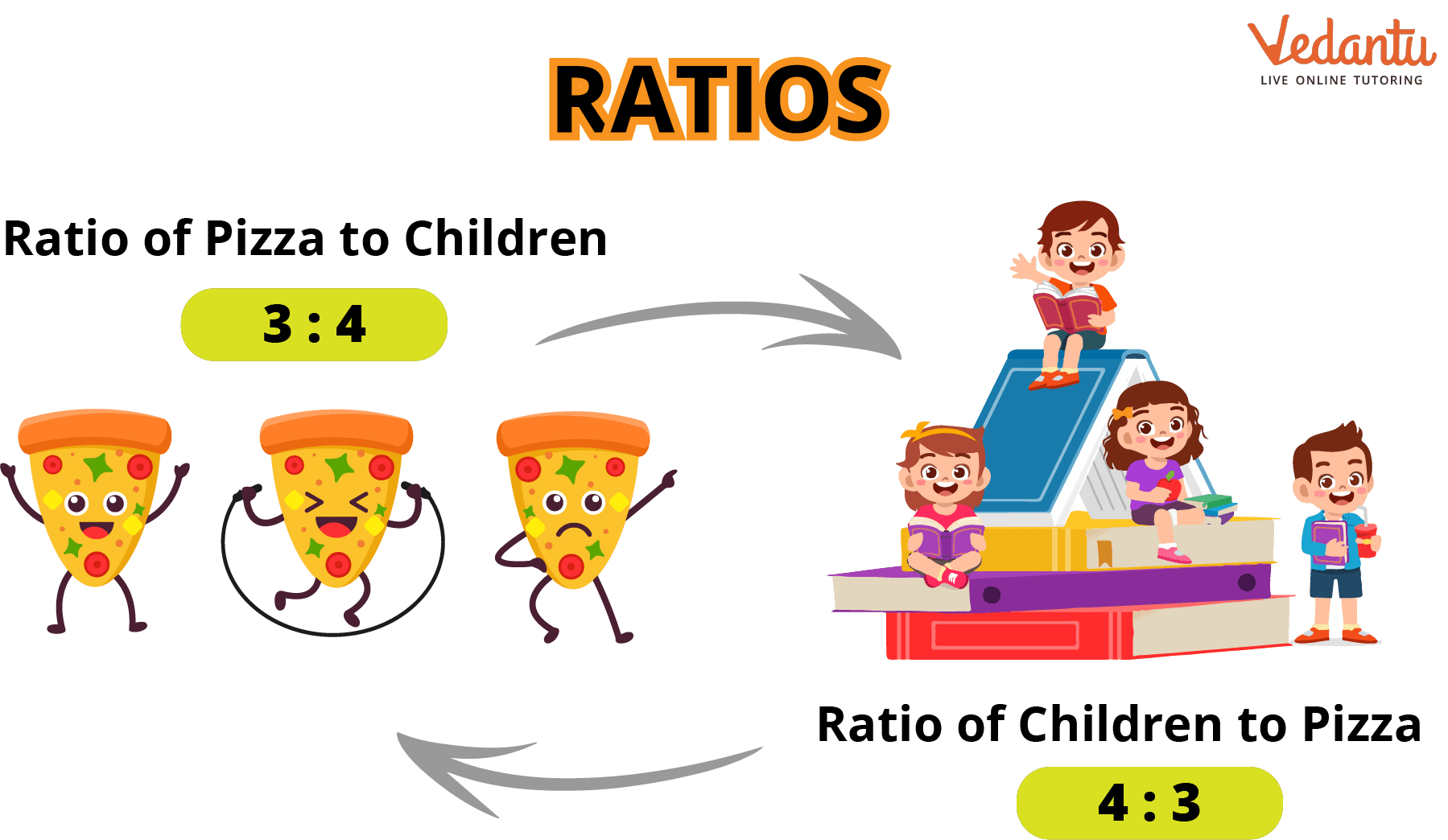Courses
Courses for Kids
Free study material
Free LIVE classes
More

# How to Solve Ratios?LIVE
Join Vedantu’s FREE Mastercalss

## A Brief on How to Solve Ratios!

The comparison of two quantities by division is very efficient in several situations. As the simplified representation of two quantities of the same type, a ratio can be defined as. The ratio of one quantity to another gives the number of times one is equal to the other. A ratio can be defined as the number that represents one quantity as a fraction of another. We can only compare two numbers in a ratio if they have the same unit and the sign that we use to denote a ratio is “:”. In a fraction, it is written using “/” and we also use “to” to represent a ratio.

## Proportion and Ratio

A ratio is known as the division of two quantities. It is usually stated as "x is to y," although it can alternatively be written as "$x:y$" or "$\dfrac{x}{y}$".

A proportional equation states that two ratios are equivalent in comparison. A ratio is written as $x: y:: z: w$ and means that x is equivalent to y as z is to w because w and y are not equal to zero,$\dfrac{x}{y}$ equals $\dfrac{z}{w}$.

## What is Ration (Ratio)?

If a and b are two values of the same type and with the same units, b is not equal to 0. The quotient $\dfrac{a}{b}$ is referred to as the ratio between a and b. The colon symbol represents ratios. The ratio $\dfrac{a}{b}$ has no units and is expressed as a: b.

## Uses of Ratio

A ratio is a method of comparing two numbers of the same type or demonstrating a relationship.

Ratios are used to compare similar goods. For example, we may use a ratio to compare the number of boys to girls in your class. Another example would be calculating the proportion of peanuts to all other nuts in a jar of mixed nuts.

Ratios are expressed in various ways, all of which have the same meaning. Here are several examples of how to write the number of boys and girls ratios, abbreviated as B and G . The ratio of B to G is denoted by B: G.

## Absolute Values and Reducing Ratios

In the preceding cases, we use absolute values. Ratios can be reduced by reducing them to their primary form. If you know how to reduce fractions, you can lower ratios.

A 3:15 ratio was chosen, represented by the fraction $\dfrac{3}{15}$, and simplified to 1:5 as a fraction because $3 \times 5$ is 15. This is the same as at 3:15.

The second ratio was 8:4. Which can be represented by the fraction $\dfrac{8}{4}$. The proportion can be reduced to 2:1. The ratio is the same as before, but it has been simplified for clarity.

## Problems on RatioThere are 3 students with blue eyes, 8 with brown eyes, and 4 with green eyes in a class of 15 total students. These are to be found:

a. What percentage of the class's students have blue eyes?

Solution:

Three children have blue eyes. There are 15 children.

Ratio: 3:15

b. How many children have green eyes as opposed to brown eyes?

Solution:

8 children have brown eyes. 4 children have green eyes.

Ratio: 8:4

## Conclusion

A ratio defines the relationship between the amounts of two or more objects. This method is used to compare quantities of the same type. When two or more ratios are equal, they are proportionate. This can be represented in the equals sign or the colon symbol in two ways. As in a:b::c:d or $\dfrac{a}{b}$= $\dfrac{c}{d}$. The ratio remains unchanged if we multiply or divide each term in the ratio by the same number, the ratio remains unchanged. If any three portions have the same ratio between the first and second quantities, so the three portions are in continuing proportion.

Last updated date: 21st Sep 2023
Total views: 91.5k
Views today: 0.91k

## FAQs on How to Solve Ratios?

1. What are the differences between ratio and proportion?

The most basic differences between ratio and proportion are discussed below:

 Ratio Proportion We use ratios to compare two things that share the same unit. We use proportion because it allows us to check the equality of two ratios. We use a (:) or a (/) to represent a ratio. We use a (::) double-colon or a (=) equal sign to represent a proportion. A ratio is an expression. A proportion is an equation. It offers to describe the quantitative relationship between two things. It offers to describe the quantitative relationship between two things with a total. The keyword to identify a ratio in a problem is “to every”. The keyword to identify a proportion in a problem is “out of”.

2. Can we represent ratios using fractions?

Yes, we can represent ratios using fractions. 7:9 can be written as  $\dfrac{7}{9}$, for example.

3. Why are ratios important?

Ratios are essential because they allow us to express quantities in a way that is easier to interpret. It is used to compare the size of two or more quantities for each other.